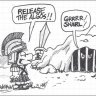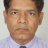# Combining Lower Indicators on One Window?#### J007RMC

##### Active member
2019 Donor
VIP
Ive combined an FP_HRP, Ironrod, hilo and the hull pack. Should I expect problems by placing these 4 indicators into one lwr window? Im sure hoping no issues arise.Really my goal is to maximize laptop screen space perhaps the hull is not needed because I do run a tmo. I cannot share due to proprietary indicators onboard by RobertLast edited:#### J007RMC

##### Active member
2019 Donor
VIP
Well Ive decided to run just one indicator in the single window but still I am curious as to my original question#### markos

##### Well-known member
VIP
@J007RMC Unless I am mistaken, you can just open the studies panel and physically drag your lower into the TMO space. With luck they will coexist.
That's all I do, just click and drag one lower into another's box. Good luck, Markos

•J007RMC#### J007RMC

##### Active member
2019 Donor
VIP
Markos Ill try this running the tmo and lower ironrod studies s in same lower window. Well I trade on a laptop so I'm always looking for ways to decrease my indicator space and enlarge m trading charts space. I usually run two charts at a time.

Last edited:
T

#### tomsk

##### Well-known member
VIP
Ive combined an FP_HRP, Ironrod, hilo and the hull pack. Should I expect problems by placing these 4 indicators into one lwr window? Im sure hoping no issues arise.

@J007RMC Unless I am mistaken, you can just open the studies panel and physically drag your lower into the TMO space. With luck they will coexist.
That's all I do, just click and drag one lower into another's box. Good luck, Markos

@J007RMC @markos In so combining such studies, one of the common anomalies is that the scaling in all these studies may be different, so that is why many such studies are normalized to a standard scale when they are combined. All the best!

Last edited:
•markos and J007RMC2019 Donor
VIP
I see thnaks

D

#### dolomick

##### Member
@markos I have an unanswered request to normalize some studies and looked at the one example posted about how to do it, but I am still not good enough to fully understand. The example was given with a MACD study. Can you or someone post or link to more examples or tutorials, and/or perhaps normalize the studies I requested here, so I can learn to do it myself in the future?

Thanks.#### markos

##### Well-known member
VIP
I have a number of examples. Don't get caught in the weeds. Take pieces out of a script and build something that interests you.
Follow up tomorrow, Hopefully I'll have a little more time. The following codes are from our Onenote in the Tutorial section.
Load these up. Some are good indicators and CSA's just as they are. Remember to put a (#) in front of a line in the editor to see what it does.
Markos
Code:
``````08:49 JohnnyQuotron: Good morning thinkorswim.  I was working through the Snippets again over the weekend and came upon a question.
https://tos.mx/Z1lBBy
(I don't know what happened to the missing sentence below)
will illustrate I hope.
The input portion of the uppermost subchart is as stanL wrote it,

script normalizePlot
{
input data = close;
input newRngMin = -1; # Replaced later by 0
input newRngMax = 1;  #Replaced later by 100
def HHData = HighestAll( data );
def LLData = LowestAll( data );
plot normalizedRange = ((( newRngMax - newRngMin ) * ( data - LLData )) / ( HHData - LLData )) + newRngMin;
}``````
Code:
``````12:32 Paris: Here's an example from BLT several years ago that normalizes plots.

# Normalized Plots
# ZZZ
# 05.30.2015
# Chat room request from Moneymiser21

# Plot the following:
#
# (A) Standard MACD line (12, 26, 9), no histogram, no signal line.
# (B) Vertical line whenever the MACD shows positive divergence from ADX(14)
#
# ADX by itself tells you strength of trend but not the direction of trend.
# It might be best to include DI+/DI- to know if it is truly divergent.
#
# While implementation of this using normalization of plots may not be perfect,
# it is close to the relationships of the actual indicators. This study includes
# the MACD.value, DI+, DI- and ADX.
#
# On the daily SPX we can see that there are a couple of places where DI- peaks
# and starts falling (bullish) while MACD is still falling (bearish). These
# types of divergences are not seen with ADX alone. With all of the plots in
# one study, it will be easier for one to see divergences.

declare lower;

script normalizePlot {
input data = close;
input newRngMin = -1;
input newRngMax = 1;
def hhData = HighestAll( data );
def llData = LowestAll( data );
plot nr = ((( newRngMax - newRngMin ) * ( data - llData )) / ( hhData - llData )) + newRngMin;
}

input newRngMax  = 100;
input newRngMin  = 0;

plot newMACDplot = normalizePlot(macd().value, newRngMin, newRngMax);
plot newDIplus   = normalizePlot(diPlus(), newRngMin, newRngMax);
plot newDIminus  = normalizePlot(diminus(), newRngMin, newRngMax); ``````
Code:
``````12:37 Mobius: Adam - Here is your studies Normalized

# Normalized Ehlers Stochastic and AMA
# Mobius
# Chat Room Request 03.21.2018

declare lower;

input price = close;
input length = 20;
input cutoffLength = 10;
input over_bought = 0.8;
input over_sold = 0.2;
input mode = {default Predictive, Conventional};

def filt = reference EhlersRoofingFilter(price, cutoffLength);
def highestP = Highest(filt, length);
def lowestP = Lowest(filt, length);
def stoch = if (highestP - lowestP) != 0 then (filt - lowestP) / (highestP - lowestP) else 0;

plot Stochastic = reference EhlersSuperSmootherFilter(stoch, cutoffLength);
plot OverBought = over_bought;
plot OverSold = over_sold;
plot Sell;

switch (mode) {
case Predictive:
Buy = if Stochastic crosses below OverSold then OverSold + 0.05 else Double.NaN;
Sell = if Stochastic crosses above OverBought then OverBought - 0.05 else Double.NaN;

case Conventional:
Buy = if Stochastic crosses above OverSold then OverSold + 0.05 else Double.NaN;
Sell = if Stochastic crosses below OverBought then OverBought - 0.05 else Double.NaN;
}

Stochastic.SetDefaultColor(GetColor(5));

OverBought.SetDefaultColor(GetColor(7));
OverSold.SetDefaultColor(GetColor(7));

Sell.SetDefaultColor(Color.DOWNTICK);
Sell.SetPaintingStrategy(PaintingStrategy.ARROW_DOWN);
Sell.HideBubble();

script Scale {
input c = close;
input Min = 0;
input Max = 1;
def hh = Highest(c, 20);
def ll = Lowest(c, 20);
plot Range = (((Max - Min) * (c - ll)) /  (hh - ll)) + Min;
}

input fastLength = 2;
input slowLength = 30;
input effRatioLength = 10;

assert(fastLength > 0, "'fast length' must be positive: " + fastLength);
assert(slowLength > 0, "'slow length' must be positive: " + slowLength);

def direction = AbsValue(price - price[effRatioLength]);
def volatility = sum(AbsValue(price - price), effRatioLength);
def ER = if volatility != 0 then direction / volatility else 0;
def FastSF = 2 / (fastLength + 1);
def SlowSF = 2 / (slowLength + 1);
def ScaledSF = ER * (FastSF - SlowSF) + SlowSF;
def AMA = compoundValue(1, AMA + Sqr(ScaledSF) * (price - AMA), price);

# End Code Normalized Study ``````
Code:
``````---- Monday, August 13, 2018 -------
07:43 Nube: Because there can never be enough oscillators
#
# Implied Move Oscillator
# Nube 8.13.18
# close in relation to Implied Move Bands

declare lower;
input Duration  = {Default "30 Days", "90 Days"};
def c  = close;

script normalize {
input h = high;
input l = low;
input c = close;
input Length = 20;
input AvgPeriod = 2;
def ll    = Lowest(l, Length);
def cll   = c - ll;
def hhll  = Highest(h, Length) - ll;
def norm  = if   hhll != 0
then Average(cll / hhll, AvgPeriod)
else norm;
plot
n = norm;
}

def days;
Switch (Duration) {
case "30 Days":
days      = 30;
case "90 Days":
days      = 90;

}
def ImpliedMoves = 1.0;
def day = AggregationPeriod.DAY;
def ap  = GetAggregationPeriod();
def iv  = if   IsNaN(imp_volatility())
then iv
else imp_volatility();

def ImpliedMove  = c * iv * Sqrt(days/(day/ap) / 365);
def upperBand = c + ImpliedMove * ImpliedMoves;
def lowerBand = c - ImpliedMove * ImpliedMoves;
def ivRange = normalize(hi, lo, c, 1);

plot
Range = ivRange;
Range.  AssignValueColor(if   ivRange < ivRange
or   ivRange == 0
then CreateColor(200,50,50)
else CreateColor(50,200,50));

plot
Mid = .5;
Mid.SetDefaultColor(Color.Gray);
Mid.SetStyle(Curve.Long_Dash);
# f/ Implied Move Oscillator``````

•BenTen
T

#### tomsk

##### Well-known member
VIP
@markos Those are great scripts for normalizing ranges, I typically normalize oscillators whenever they have very different ranges. Thanks for posting.

•markosExploring Scan Combining Unusual volume and near 52 weeks high ... Playground 26